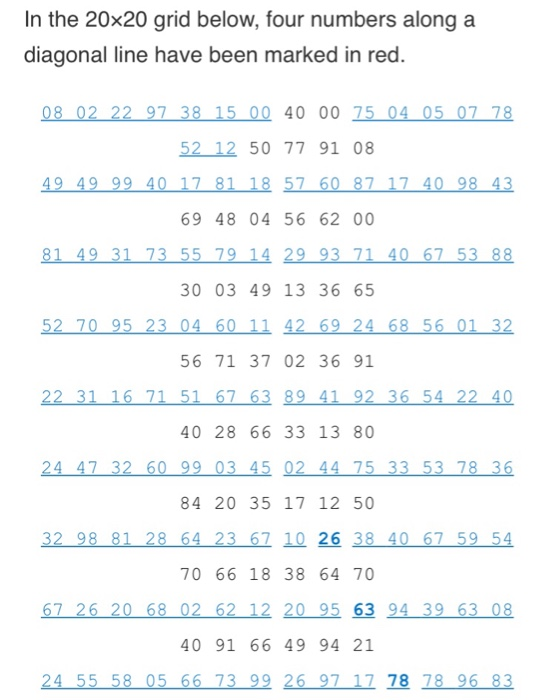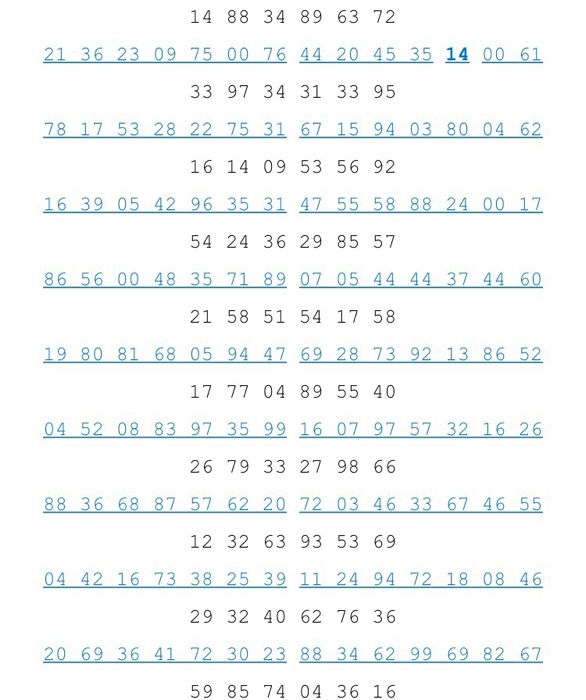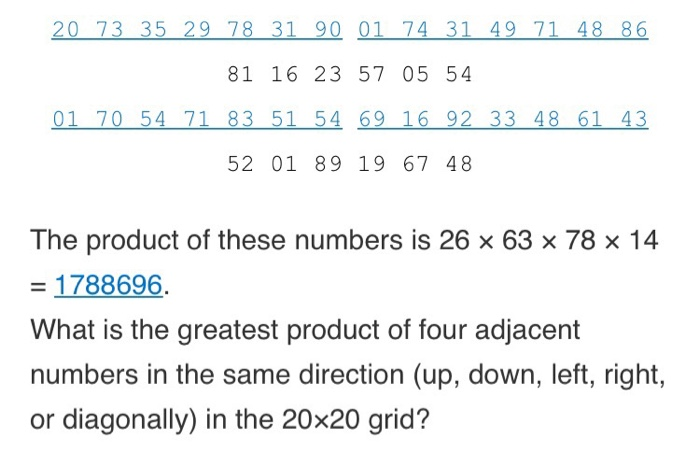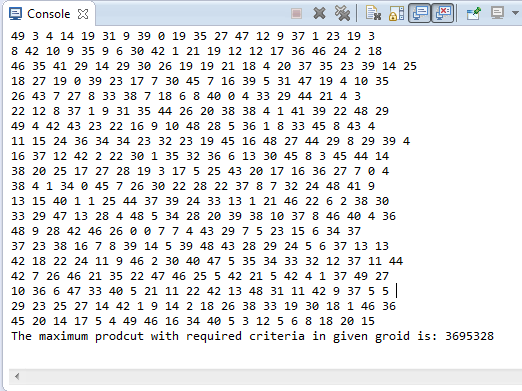# Answered! In the 20×20 grid below, four numbers along a diagonal line have been marked in red. …

Using Java to write a program that calculates the following :In the 20×20 grid below, four numbers along a diagonal line have been marked in red.

import java.util.Random;

public class findMaxProduct {

Don't use plagiarized sources. Get Your Custom Essay on
Answered! In the 20×20 grid below, four numbers along a diagonal line have been marked in red. …
GET AN ESSAY WRITTEN FOR YOU FROM AS LOW AS \$13/PAGE

public static long findMaximumProduct(int grid[][]) {
long maxProduct = 0;

for(int r=0; r < grid.length; r++) {

for(int c=0; c < grid[r].length; c++) {
long product;
// Check for horizontal row of 4 elements starting from this
if(grid[r].length > c+3) {
product = grid[r][c] * grid[r][c+1] * grid[r][c+2] * grid[r][c+3];
if(product > maxProduct)
maxProduct = product;
}

// Check for vertical col of 4 elements starting from this
if(grid.length > r+3) {
product = grid[r][c] * grid[r+1][c] * grid[r+2][c] * grid[r+3][c];
if(product > maxProduct)
maxProduct = product;
}

// Check for diagonal from top left to bottom right starting from this
if(grid.length > r+3 && grid[r].length > c+3) {
product = grid[r][c] * grid[r+1][c+1] * grid[r+2][c+2] * grid[r+3][c+3];
if(product > maxProduct)
maxProduct = product;
}

// Check for diagonal from top left to bottom right starting from this
if(0 < (r-3) && (c-3) > 0) {
product = grid[r][c] * grid[r-1][c-1] * grid[r-2][c-2] * grid[r-3][c-3];
if(product > maxProduct)
maxProduct = product;
}
}
}
return maxProduct;
}

public static void main(String[] args) {
int grid[][] = new int;
Random r = new Random();
System.out.println(“Grid is: “);
for(int i=0; i<20; i++) {
for(int j=0; j<20; j++) {
grid[i][j] = r.nextInt(50);
System.out.print(grid[i][j] + ” “);
}
System.out.println();
}

System.out.println(“The maximum prodcut with required criteria in given grid is: ” + findMaximumProduct(grid));
}

}